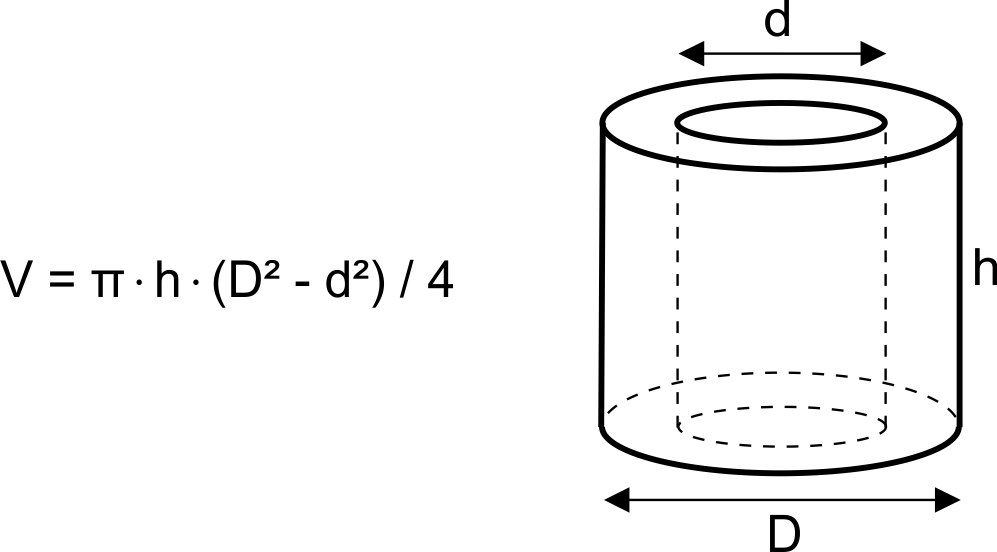# Cylinder Volume Calculator

Created by Luis Hoyos
Last updated: Feb 18, 2023

If you are searching for how to find the volume of a cylinder, this cylinder volume calculator is for you.

The volume of a cylinder is a quantity present in many applications. For example, in a vehicle, the engine displacement term refers to the volume swept by the piston in the engine cylinders, multiplied by the number of cylinders.

This tool calculates the cylinder volume in a matter of seconds. Still, in the following sections, we present the cylinder volume formula to do it manually, including the equation to calculate the volume of a hollow cylinder.

To know more about the different volume units, you can look at our volume converter.

## Solid cylinder volume formula

Like all regular prisms, the volume of a cylinder is the surface area of an end multiplied by its length (the same happens for the volume of a rectangular prism). Therefore, the formula to calculate the volume of a cylinder (solid) is:

$V = \text{π}\times R^2 \times h$

, where:

• $V$ — Cylinder volume;
• $R$ — Cylinder radius; and
• $h$ — Cylinder height.

We can express the previous formula **in terms of the cylinder diameter ($D$), remembering that $D = 2R$:

$V = \frac{\text{π}}{4}\times D^2 \times h$

## Formula to calculate the volume of a hollow cylinder

The equation to calculate the volume of a hollow cylinder is:

$V = \text{π}\times (R^2 - r^2)\times h$

• $V$ — Cylinder volume;
• $R$ — Cylinder external radius;
• $r$ — Cylinder internal radius; and
• $h$ — Cylinder height.

We can also express the previous formula in terms of the external ($D) and internal diameters ($d):

$V = π\times (D^2 - d^2)\times h/4$

## How to use this cylinder volume calculator

For a solid cylinder:

1. Input the height of the cylinder in the "Height" box. Suppose it equals 20 cm.
2. Input the radius of the cylinder in the "Radius" box. Let's say it equals 5 cm.
3. The cylinder volume should be 1570.8 cm3. That's how you find the volume of a cylinder with this calculator.

How to calculate the volume of a hollow cylinder:

1. Input the height of the cylinder in the "Height" box. Suppose it equals 20 cm.
2. Input the external diameter of the cylinder in the "Diameter external" box. Let's say it equals 10 cm.
3. Input the internal diameter of the cylinder in the "Diameter internal" box. Let's say it equals 5 cm.
4. The cylinder volume should be 1178.1 cm3. That's how you calculate the volume of a hollow cylinder with this tool.
Luis Hoyos
Right / oblique full cylinder
Height (h)
in
in
Diameter (D)
inVolume (V)
cu in
Hollow cylinder
Height (h)
in
External diameter (D)
in
Internal diameter (d)
inVolume
cu in
People also viewed…

### Rectangular prism

The rectangular prism calculator can obtain the length, width, height, surface area, volume, and diagonal of any rectangular prism!